# 02_housing-exploratory-data-analysis

## Portland housing data - exploratory data analysis¶¶

In this second notebook, we use the cleaned and filtered data produced from notebook 1. Here we explore the spatial distribution of our dataset.

So far, we worked with pandas `DataFrame` objects, to map the properties, we import the ArcGIS API for Python. This adds a `GeoAccessor` and spatially enables your `DataFrame` objects. As you will see in this notebook, you can then easily plot your data both as charts and as maps.

In :
```import pandas as pd
import matplotlib.pyplot as plt
%matplotlib inline

from arcgis.gis import GIS
from arcgis.features import GeoAccessor, GeoSeriesAccessor
gis = GIS()
```

In :
```csv_path = 'resources/houses_for_sale_filtered.csv'
```
Out:
Unnamed: 0 SALE TYPE PROPERTY TYPE ADDRESS CITY STATE ZIP PRICE BEDS BATHS YEAR BUILT DAYS ON MARKET PRICE PER SQFT HOA PER MONTH STATUS URL SOURCE MLS LATITUDE LONGITUDE
0 3 MLS Listing Mobile/Manufactured Home 6112 SE Clatsop St Portland OR 97206.0 35000.0 2.0 1.0 1981.0 93.0 44.0 0.0 Active http://www.redfin.com/OR/Portland/6112-SE-Clat… RMLS 18331169 45.461282 -122.600153
1 8 For-Sale-by-Owner Listing Single Family Residential 6901 SE Oaks Park Way Slip 32 Portland OR 97202.0 60000.0 1.0 1.0 1939.0 359.0 55.0 0.0 Active http://www.redfin.com/OR/Portland/6901-SE-Oaks… Fizber.com 4933081 45.474369 -122.662307
2 14 For-Sale-by-Owner Listing Mobile/Manufactured Home 11187 SW Royal Villa Dr Portland OR 97224.0 69950.0 2.0 2.0 1976.0 44.0 47.0 0.0 Active http://www.redfin.com/OR/Portland/11187-SW-Roy… Fizber.com 4974567 45.398486 -122.796175

3 rows × 23 columns

In :
```prop_df.shape
```
Out:
`(3624, 23)`
In :
```prop_df.columns
```
Out:
```Index(['Unnamed: 0', 'SALE TYPE', 'PROPERTY TYPE', 'ADDRESS', 'CITY', 'STATE',
'ZIP', 'PRICE', 'BEDS', 'BATHS', 'LOCATION', 'SQUARE FEET', 'LOT SIZE',
'YEAR BUILT', 'DAYS ON MARKET', 'PRICE PER SQFT', 'HOA PER MONTH',
'STATUS', 'URL', 'SOURCE', 'MLS', 'LATITUDE', 'LONGITUDE'],
dtype='object')```

Drop redundant columns

In :
```try:
prop_df.drop(columns=['Unnamed: 0'], inplace=True)
prop_df.drop(columns=['Unnamed: 0.1'], inplace=True)
except:
pass
```

### Visualize spatially¶¶

Convert to Spatially Enabled DataFrame

In :
```prop_sdf = pd.DataFrame.spatial.from_xy(prop_df, 'LONGITUDE','LATITUDE')
type(prop_sdf)
```
Out:
`pandas.core.frame.DataFrame`
In [ ]:
```pdx_map = gis.map('Portland, OR')
pdx_map.basemap = 'streets'
pdx_map
```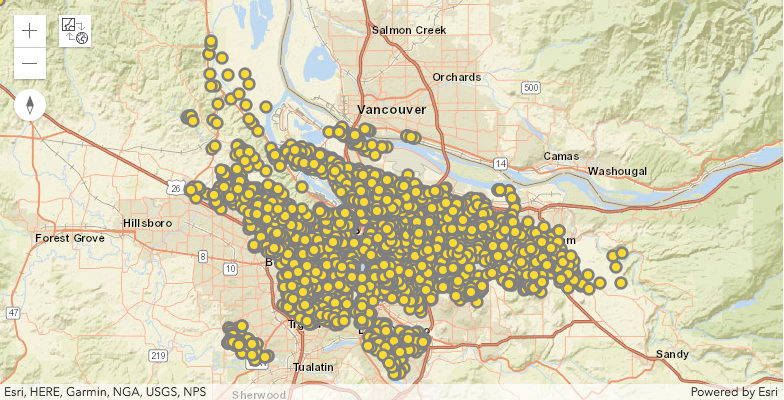In :
```prop_sdf.spatial.plot(map_widget=pdx_map)
```
Out:
`True`

#### Explore density of properties for sale¶¶

You can render the same data using a heatmap renderer, which visualizes the spatial density of the properties (houses) using a heatmap.

In [ ]:
```pdx_density_map = gis.map('Portland, OR')
pdx_density_map.basemap='gray'
pdx_density_map
```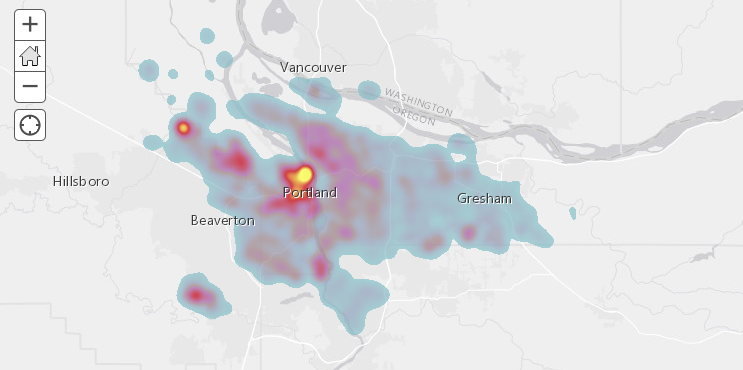In :
```prop_sdf.spatial.plot(map_widget=pdx_density_map, renderer_type='h')
```
Out:
`True`

There is a hotspot near downtown Portland. There are few other isolated hotspots around the downtown as well. These could be pockets of communities that are spread around a major city.

### Visualize geographic distributions of housing properties¶¶

Using different renderers such as ‘class colored renderer’, you can visualize and investigate if there is any spatial phenomena observable for the following columns.

• How is property age spatially distributed?
• Does property price drop outward from city center?
• Does property size increase in suburbs?
• hoa vs no hoa

#### Visualize spatial distribution by property age¶¶

In [ ]:
```pdx_age_map = gis.map("Portland, OR")
pdx_age_map.basemap = 'gray-vector'
pdx_age_map
```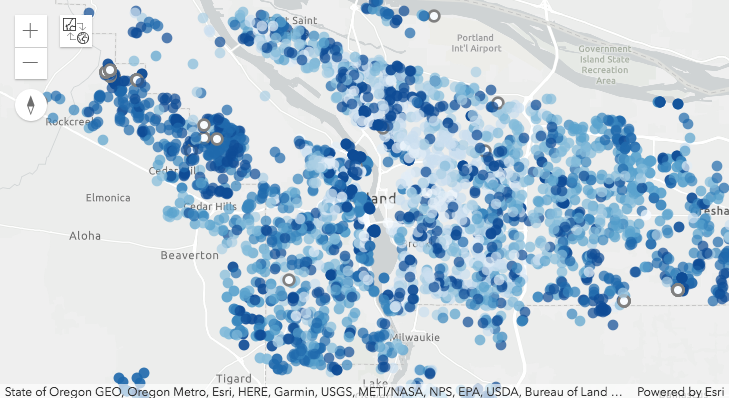In :
```prop_sdf.spatial.plot(map_widget = pdx_age_map,
renderer_type='c', # for classs breaks renderer
method='esriClassifyNaturalBreaks',  # classification scheme
class_count=10,  # between 1900 - 2000, each decade in a class
col='YEAR BUILT',
cmap='Blues',  # matplotlib color map
alpha=0.7,
outline_color=[0,0,0,0])
```
Out:
`True`

If you notice the syntax above, it mirrors the general syntax of plotting a DataFrame as a chart. It uses the same color map and symbols of `matplotlib`. Internally, the ArcGIS API for Python converts this syntax to ArcGIS symbology and renders it on a map.

Since the colormap and symbols is that of `matplotlib`, you can collect the class breaks from the map above and use that to plot the same data as a bar chart as shown below. Combining the map and chart gives you a powerful way to interactively explore the spatial and statistical distribution of your dataset.

In :
```age_class_breaks = pdx_age_map.layers.layer.layerDefinition.drawingInfo.renderer.classBreakInfos
# print(len(age_class_breaks))
cbs_list = []
cmap_list = []
for cb in age_class_breaks:
#     print(cb.description)  # print the class break labels
cbs_list.append(cb.classMaxValue)
cmap_list.append([x/255.0 for x in cb.symbol.color])

# build a histogram for the same class breaks
n, bins, patches = plt.hist(prop_sdf['YEAR BUILT'], bins=cbs_list)

# apply the same color for each class to match the map
idx = 0
for c, p in zip(bins, patches):
plt.setp(p, 'facecolor', cmap_list[idx])
idx+=1

plt.title('Histogram of YEAR BUILT column')
```
Out:
`Text(0.5,1,'Histogram of YEAR BUILT column')`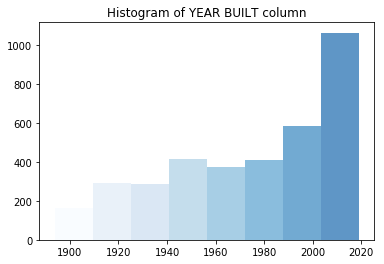#### Visualize spatial distribution by price¶¶

In [ ]:
```pdx_price_map = gis.map("Portland, OR")
pdx_price_map.basemap = 'gray-vector'
pdx_price_map
```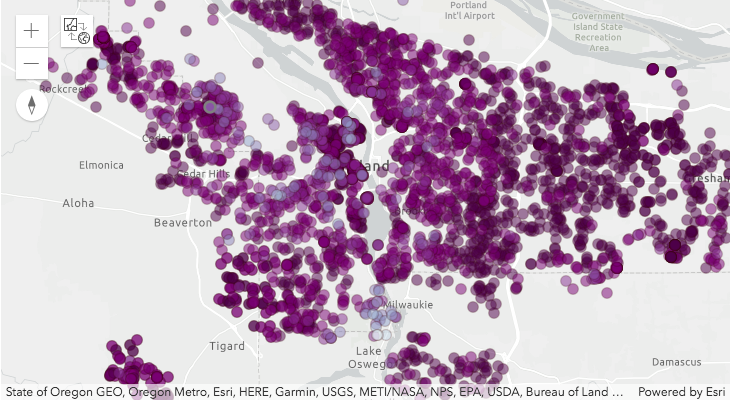In :
```prop_sdf.spatial.plot(map_widget = pdx_price_map,
renderer_type='c', # for classs breaks renderer
method='esriClassifyQuantile',  # classification scheme
class_count=10,  # between 1900 - 2000, each decade in a class
col='PRICE',
cmap='BuPu_r',  # matplotlib color map
alpha=0.5,
outline_color=[50,0,0,50], line_width=1)
```
Out:
`True`
In :
```price_class_breaks = pdx_price_map.layers.layer.layerDefinition.drawingInfo.renderer.classBreakInfos
# print(len(age_class_breaks))
cbs_list = []
cmap_list = []
for cb in price_class_breaks:
#     print(cb.description)  # print the class break labels
cbs_list.append(cb.classMaxValue)
cmap_list.append([x/255.0 for x in cb.symbol.color])

# build a histogram for the same class breaks
n, bins, patches = plt.hist(prop_sdf['PRICE'], bins=cbs_list)

# apply the same color for each class to match the map
idx = 0
for c, p in zip(bins, patches):
plt.setp(p, 'facecolor', cmap_list[idx])
idx+=1

plt.title('Histogram of PRICE column')
```
Out:
`Text(0.5,1,'Histogram of PRICE column')`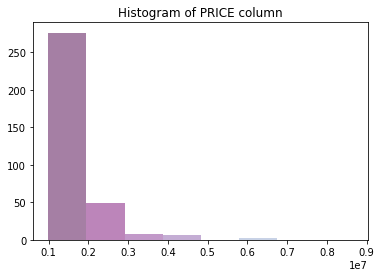#### Visualize spatial distribution of property size¶¶

In [ ]:
```pdx_size_map = gis.map("Portland, OR")
pdx_size_map.basemap = 'gray-vector'
pdx_size_map
```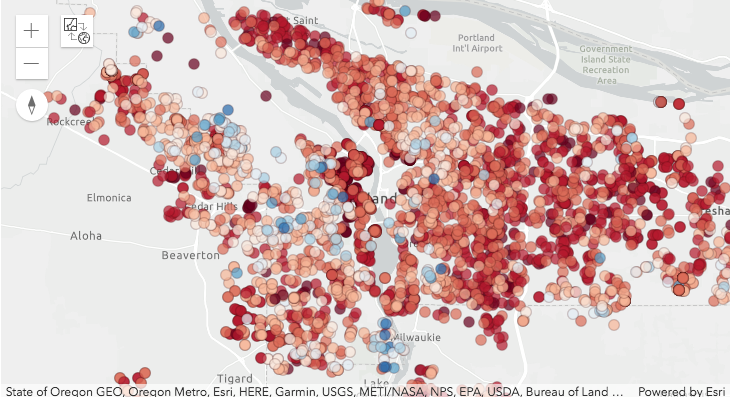In :
```prop_sdf.spatial.plot(map_widget = pdx_size_map,
renderer_type='c', # for classs breaks renderer
method='esriClassifyNaturalBreaks',  # classification scheme
class_count=10,  # between 1900 - 2000, each decade in a class
col='SQUARE FEET',
cmap='RdBu',  # matplotlib color map
alpha=0.7,
outline_color=[50,0,0,50], line_width=1)
```
Out:
`True`
In :
```size_class_breaks = pdx_size_map.layers.layer.layerDefinition.drawingInfo.renderer.classBreakInfos
# print(len(age_class_breaks))
cbs_list = []
cmap_list = []
for cb in size_class_breaks:
#     print(cb.description)  # print the class break labels
cbs_list.append(cb.classMaxValue)
cmap_list.append([x/255.0 for x in cb.symbol.color])

# build a histogram for the same class breaks
n, bins, patches = plt.hist(prop_sdf['SQUARE FEET'], bins=cbs_list)

# apply the same color for each class to match the map
idx = 0
for c, p in zip(bins, patches):
plt.setp(p, 'facecolor', cmap_list[idx])
idx+=1

plt.title('Histogram of SQUARE FEET column')
```
Out:
`Text(0.5,1,'Histogram of SQUARE FEET column')`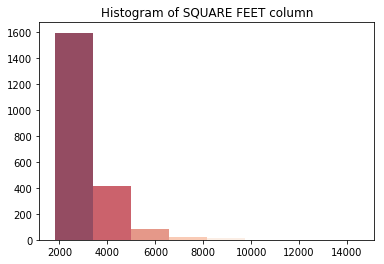#### Spatial distribution of HoA¶¶

In [ ]:
```pdx_hoa_map = gis.map("Portland, OR")
pdx_hoa_map.basemap = 'gray-vector'
pdx_hoa_map
```In :
```#plot properties without HOA as hollow
# prop_sdf_hoa_f = prop_df[prop_df['HOA PER MONTH']==0]
# prop_sdf_hoa_f.spatial.plot(map_widget=pdx_hoa_map, symbol_type='simple',
#                             symbol_style='+',outline_color='Blues',
#                             marker_size=7)

prop_sdf_hoa_2 = prop_df[prop_df['HOA PER MONTH']>0]

prop_sdf_hoa_2.spatial.plot(map_widget = pdx_hoa_map,
renderer_type='c', # for classs breaks renderer
method='esriClassifyQuantile',  # classification scheme
class_count=10,  # between 1900 - 2000, each decade in a class
col='HOA PER MONTH',
cmap='RdBu',  # matplotlib color map
alpha=0.7,
outline_color=[0,0,0,0], line_width=0)
```
```/Users/atma6951/anaconda3/envs/geosaurus_gold/lib/python3.6/site-packages/arcgis/features/geo/_accessor.py:861: SettingWithCopyWarning:
A value is trying to be set on a copy of a slice from a DataFrame.
Try using .loc[row_indexer,col_indexer] = value instead

See the caveats in the documentation: http://pandas.pydata.org/pandas-docs/stable/indexing.html#indexing-view-versus-copy
self._data[col] = self._data[col]
/Users/atma6951/anaconda3/envs/geosaurus_gold/lib/python3.6/site-packages/arcgis/features/geo/_accessor.py:1968: SettingWithCopyWarning:
A value is trying to be set on a copy of a slice from a DataFrame.
Try using .loc[row_indexer,col_indexer] = value instead

See the caveats in the documentation: http://pandas.pydata.org/pandas-docs/stable/indexing.html#indexing-view-versus-copy
self._data['OBJECTID'] = list(range(1, self._data.shape + 1))
```
Out:
`True`
In :
```hoa_class_breaks = pdx_hoa_map.layers.layer.layerDefinition.drawingInfo.renderer.classBreakInfos
# print(len(age_class_breaks))
cbs_list = []
cmap_list = []
for cb in hoa_class_breaks:
#     print(cb.description)  # print the class break labels
cbs_list.append(cb.classMaxValue)
cmap_list.append([x/255.0 for x in cb.symbol.color])

# build a histogram for the same class breaks
n, bins, patches = plt.hist(prop_sdf['HOA PER MONTH'], bins=cbs_list)

# apply the same color for each class to match the map
idx = 0
for c, p in zip(bins, patches):
plt.setp(p, 'facecolor', cmap_list[idx])
idx+=1

plt.title('Histogram of HOA PER MONTH column')
```
Out:
`Text(0.5,1,'Histogram of HOA PER MONTH column')`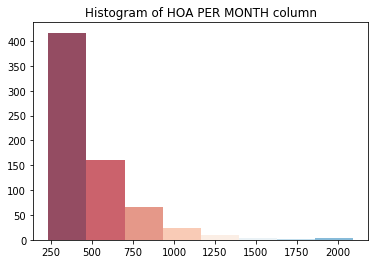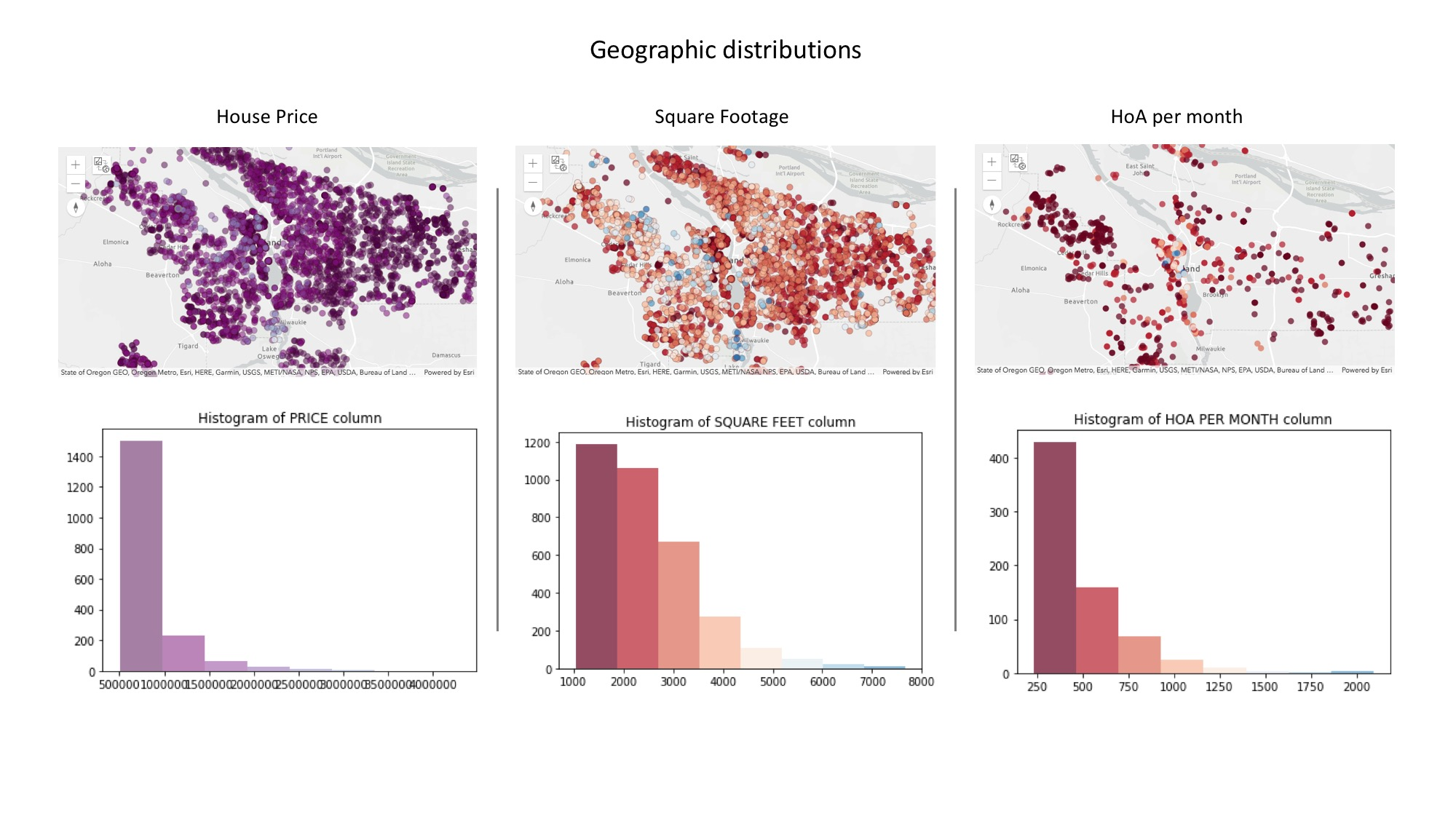### Explore distribution of numeric columns:¶¶

In :
```ax_list = prop_df.hist(bins=25, layout=(4,4), figsize=(15,12))
```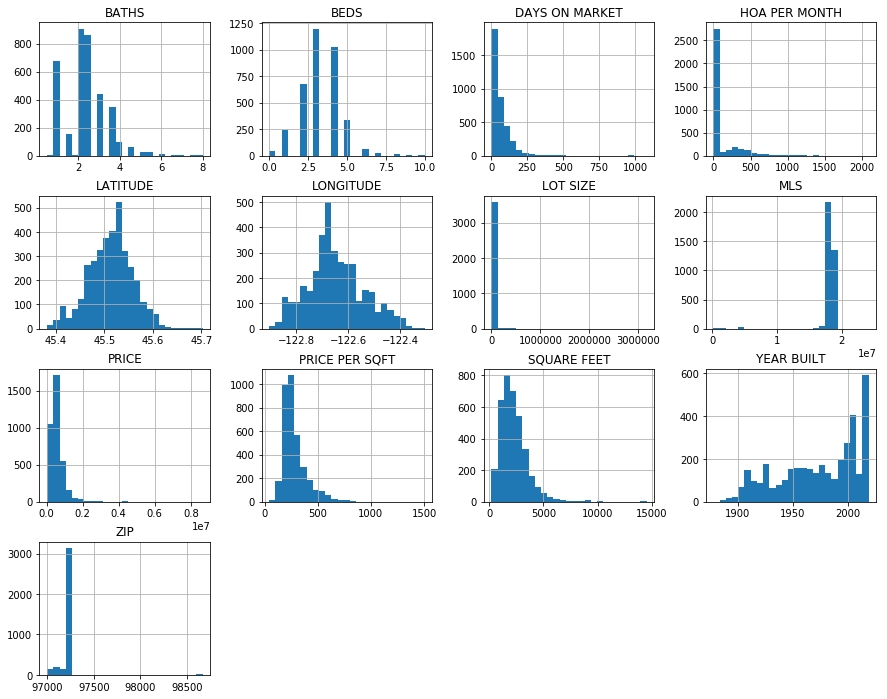Explore the frequency of categorical columns

In :
```fig2, ax2 = plt.subplots(1,2, figsize=(10,5))

prop_df['CITY'].value_counts().plot(kind='bar', ax=ax2,
title='City name frequency')
ax2.tick_params(labelrotation=45)

prop_df['PROPERTY TYPE'].value_counts().plot(kind='bar', ax=ax2,
title='Property type frequency')
ax2.tick_params(labelrotation=45)
plt.tight_layout()
```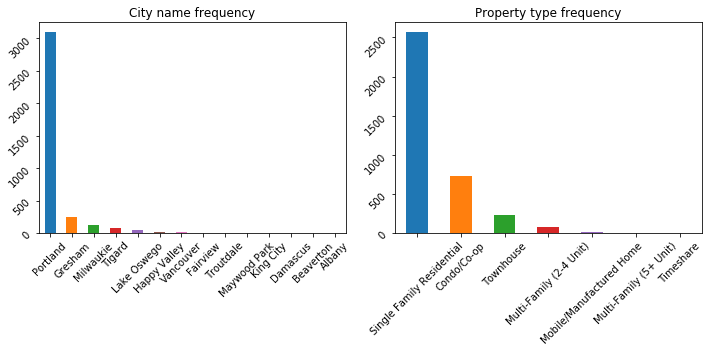### Filter based on your criteria¶¶

Let us define a few rules based on the intrinsic properties of these houses and shortlist them.

In :
```filtered_df = prop_sdf[(prop_df['BEDS']>=2) &
(prop_df['BATHS']>1)&
(prop_df['HOA PER MONTH']<=200) &
(prop_df['YEAR BUILT']>=2000) &
(prop_df['SQUARE FEET'] > 2000) &
(prop_df['PRICE']<=700000)]
filtered_df.shape
```
Out:
`(331, 23)`
In :
```(prop_sdf.shape, filtered_df.shape)
```
Out:
`((3624, 23), (331, 23))`

From `3624` houses, we shortlisted `331` of them. Below, let us visualize the statistical distribution of this shortlist.

#### Visualize statistical distribution of shortlisted properties¶¶

In :
```ax_list2 = filtered_df.hist(bins=25, layout=(4,4), figsize=(15,15))
```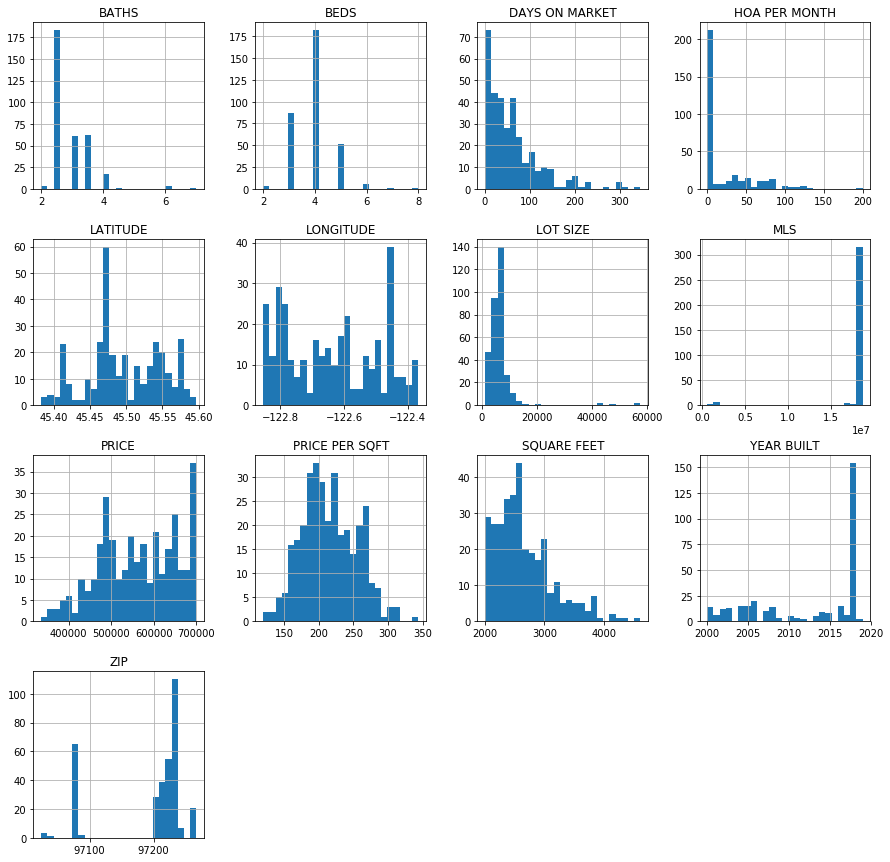From the histograms above, we notice most of the houses have `4` beds, while we requested for at least `2`. Majority of them are newly built and skewed toward upper end of the price spectrum.

#### Visualize spatial distribution of shortlisted properties¶¶

In [ ]:
```pdx_filtered_map = gis.map("Portland, OR")
pdx_filtered_map.basemap = 'gray-vector'
pdx_filtered_map
```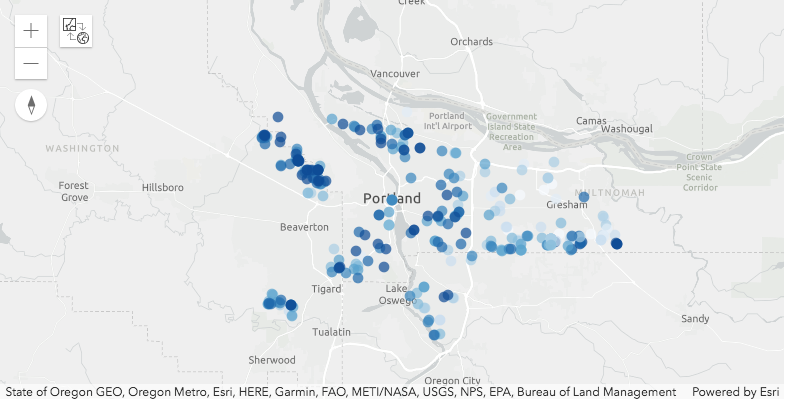In [ ]:
```filtered_df.spatial.plot(map_widget=pdx_filtered_map,
renderer_type='c',
method='esriClassifyNaturalBreaks',  # classification scheme
class_count=10,
col='PRICE',
cmap='Blues',  # matplotlib color map
alpha=0.7,outline_color=[0,0,0,0])
```

The houses in the shortlist are well spread across the Portland market. We notice spatial clustering in the distribution of property prices. Higher priced houses are mostly to the west of downtown while moderately and lower priced are spread across east and south.

#### Write the shortlisted properties to disk¶¶

So far, we used attribute queries to explore and filter out properties. We have not yet used GIS analysis to narrow them further. Before that, let us save our work to disk.

In :
```filtered_df.to_csv('resources/houses_for_sale_att_filtered.csv')
```